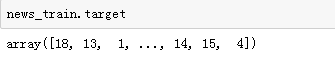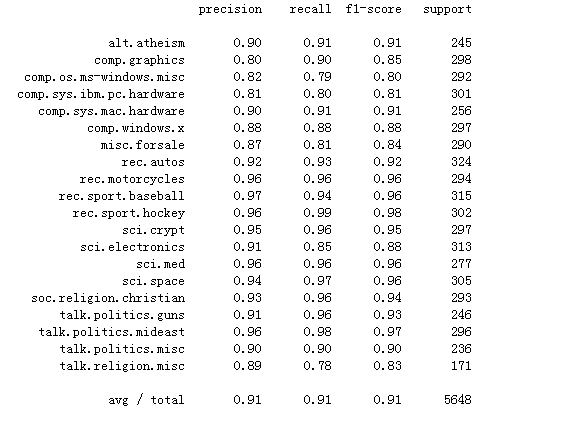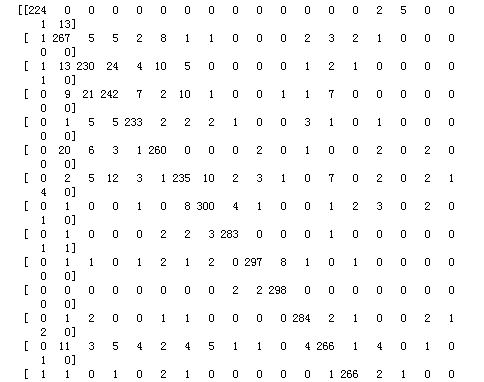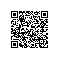# sklearn调包侠之朴素贝叶斯### 文档处理

##### TF-IDF方法

TF表示词频，对一个文档而言，词频就是词在文档出现的次数除以文档的词语总数。例如：一篇文档有1000个字，“我”字出现25次，那就是0.025；“Python”出现5次就是0.005。
IDF表示一个词的逆向文档频率指数。可以由总文档数除以包含该词出现的文档数目，然后取对数。例如：有10000个文档，“Python”只出现了10篇文章，则IDF=log(10000/10)=3；“我”字在所有文档都出现过，则IDF为0。

### 实战——文档分类

##### 数据导入和TF-IDF

from sklearn.datasets import load_filesfrom sklearn.feature_extraction.text import TfidfVectorizer

vect = TfidfVectorizer(encoding='latin-1')
X_train = vect.fit_transform(news_train.data)

##### 模型训练

from sklearn.naive_bayes import MultinomialNB
y_train = news_train.target
clf = MultinomialNB(alpha=0.0001)
clf.fit(X_train, y_train)
train_score = clf.score(X_train, y_train)

# result
# 0.99787556904400609

##### 模型评估

news_test = load_files('data/379/test')
X_test = vect.transform(news_test.data)
y_test = news_test.target

clf.score(X_test, y_test)

# result
# 0.90881728045325783


from sklearn.metrics import classification_report

pred = clf.predict(X_test)

print(classification_report(y_test, pred, target_names=news_test.target_names))from sklearn.metrics import confusion_matrix

cm = confusion_matrix(y_test, pred)

print(cm)使用钉钉扫一扫加入圈子
+ 订阅